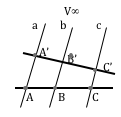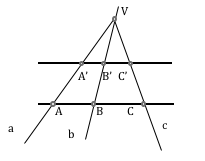# Geometría proyectiva: Ordered triples of elements

## Ordered triples of elementsThe metric geometry is based on the known Pythagorean theorem. All theorems are deduced from the concept of measurement that is derived from the right triangles.

Analogous, projective geometry is based on another important theorem, the Thales theorem, instead of a metric concept relationship establishes the concept of measurement, as projective invariant.

## Concept of triples of elements

Three elements belonging to a way of determining one notch internal.

In the case of using the elements points, then we say that three points determine a ordered triple of points.

The elements can be either straight or flat spots as, could even generate triples of hiperelementos more complex geometries.

To represent symbolically use the following notation:

• Terna points: (ABC)
• Terna straight: (abc)
• Terna plans: (abc)

The slate has a numerical value or feature associate involves the management of the terms that are.

• (ABC) = AB/AC = λ. [Ec. 1]
• (abc) = You(from)/you(and) = L. [Ec. 2]
• (abc) = You(ab)/you(ac) = L. [Ec. 3]

### Ordered triples of points

The value of an ordered list of three points as the ratio between two lengths defined, the segment formed by the first and second point of the triplet and the segment formed by the first and third points:

The segments may be signed. The direction of segment AB is contrary to the BA, or what is the same, AB = – BA

Conceptually can be understood as a triple measure of a segment on a per second.

For example, If B is the midpoint of segment AC, the triplet (ABC) = 1/2. The AC segment acts as a measurement unit.

### Ordered triples of lines

The value of the ordered list of three straight as the ratio between two defined breasts, the angle formed by the first two lines and determines the first and third straight:[Ec. 2]

Three straight beam vertex V, and three points in a series by a section of the beam line can be related by the values ​​of their shortlists.
This value is the characteristic of the triplet is a key element to classify projections, so that those in which enjoy common invariant and independent properties of the projection type.

By orthogonally projecting the points B and C the rectilinear straight rerie on the Fig.1, point B are obtained 'and C'. Triangles ABB’ and ACC 'are similar thus, applying relations by Theorem of Thales:

(abc) = You(from)/you(and) = L [Ec. 2]

The value of the sine of the angle between the lines a and b as well as that formed by lines a and c will be:

If the latter values ​​are substituted into the [Ec. 2] we:

Therefore, generally, (ABC) ≠ (abc), value from a list of lines is different from a list of points that the sectioned.

If we cut straight beam by two parallel lines not, the series are certain perspectives together, triples of points although they have the same characteristic.

One example is the tapered projection from a point V.

To be equal to the two triads is necessary that the ratio VC/VB is equal to unity. This is achieved when the vertex is an improper point, or when the lines are parallel sectioned.
This allows interesting properties in Cylindrical projections nature (improper vertex) and projections and, or, straight or flat sections.

### Conservation simple reason

When the vertex V.. straight beam is located at infinity, the term VC/VB the [Ec. 6] tends to unity, so that the list of points is equal to the triplet lines.Fig.-4 Conservation simple reason in sections parallel lines In section three lines of a beam improper vertex (parallel lines to, b and c in Figure ), different triples of points of the section results therefore have the same value or characteristic. This case corresponds to the projections known as cylindrical projections, projected in accordance with a direction orthogonal or oblique to the plane of projection, or plane of the drawing.Fig.- 5 Conservation simple reason in sections parallel lines In section three lines of a beam own vertex (parallel lines to, b and c in Figure ), different triples of points results in section therefore have the same value. This case corresponds to the conical projections on planes or lines that are parallel and in homotecias.

### Conservation simple reason in cylindrical projections:

The projective model may be useful in the study of systems of representation. Different representations for the elements are projected on a projection plane.

This process involves the use of two projective operations:

• We project point
• Sectioned the beam resulting from the projection plane.

We can use the projective terms such as to define the concept of projection of an element.

• Projecting a point from another is to define the line belongs to both elements (Rectilinear Series)
• Project a line from a point to define the plane which belongs to both elements (Make straight)
• Projecting a plane from one point to define the set of lines / planes that belongs to the point and the points / lines of the plane (Radiation from straight / flat)

By projecting the elements, The central projection may be:

• Own
• Improper

In the case of a projection with improper center (or also referred to as cylindrical projection), the simple reason is preserved in the triads of rays projecting.

(WITH) = (A’M’B’)

The projection of the midpoint, therefore, corresponds to the midpoint of the projection.

This result is useful in numerous problems in that the relationship between parts, geometry, is known.

For example obtaining projection centroid of a triangle can be limited again to locate the centroid of the triangle projected.